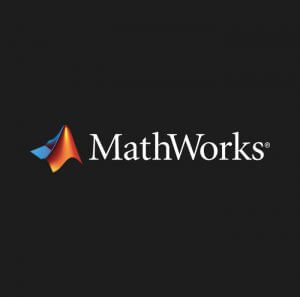Axoft

## MATLAB## MATLAB

It is a high-level language and interactive environment for numerical calculations, visualization and programming. With MATLAB, you can analyze data, develop algorithms, create models and applications. Language features, advanced tools and built-in math functions allow you to use composite problem solving methods and get an answer faster than working with tables and traditional programming languages ​​such as C / C ++ and Java.

MATLAB combines a desktop environment tuned for iterative analysis and design processes with a programming language that directly expresses the mathematics of arrays and arrays.

### Professionally built

MATLAB toolboxes are professionally designed, thoroughly tested and fully documented.

### With interactive applications

MATLAB applications allow you to see how different algorithms work with your data. Iterate until you get the results you want, then automatically create a MATLAB program to play or automate your work.

### Scalability

Scale your analyzes to run on clusters, GPUs and clouds with minor code changes. You do not need to rewrite your code or learn a large amount of data and methods out of memory.

MATLAB can be used for a wide range of tasks, including signal processing, communication systems, image and video processing, organization of control over the system, testing and measurement, financial and natural science calculations. MATLAB is used by over one million engineers and scientists at work and in scientific institutions.

Key benefits:

◊High-level programming language for numerical calculations, visualization and application development

◊Interactive tools for repetitive computing and design

Mathematical functions of linear algebra, statistics, Fourier analysis, filtering, optimization, numerical integration and solving ordinary differential equations

◊Integrated graphical data visualization tools and tools for creating custom graphs

◊Development tools to optimize code and maximize performance

◊Tools for creating applications with customizable graphical interface

◊Functions for integrating MATLAB algorithms with external applications and programming languages ​​such as C, Java, .NET and Microsoft Excel